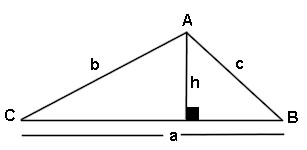# Triangle Area Angle Side Angle Calculator

This calculator requires the use of Javascript enabled and capable browsers. This calculator is designed to give the area of a triangle, assuming two angles and the side between are known, also known as ASA. Enter the two known angles and the side. Then click on calculate. In a triangle, all interior angles total to 180 degrees. No two angles can total to 180 degrees or more. It is for any shape triangle. Click on Calculate. The unknown angle, both unknown sides and the area are returned. In case you need them, here are the Trig Triangle Formula Tables, as well as the Right Triangle Angle And Side Calculator. Here is our Triangle Math Menu.

 Unit Number Required Data Entry Quantity Decimal Places Angle Units Designation degrees (decimal) radians Angle A Adjacent To Side c Angle Units Angle B Adjacent To Side c Angle Units Known Side c Length Units Calculated Results Calculated Angle C Angle Units Calculated Side a Length Units Calculated Side b Length Units Area Of Triangle Square UnitsUpdated 8.15.11

 Leave us a question or comment on FacebookSearch or Browse Our Site